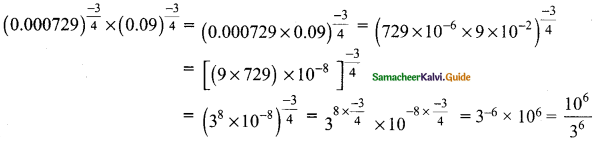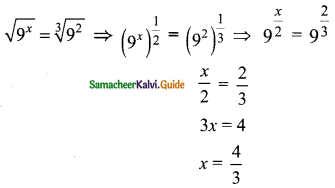Students can download Maths Chapter 2 Real Numbers Ex 2.9 Questions and Answers, Notes, Samacheer Kalvi 9th Maths Guide Pdf helps you to revise the complete Tamilnadu State Board New Syllabus, helps students complete homework assignments and to score high marks in board exams.

## Tamilnadu Samacheer Kalvi 9th Maths Solutions Chapter 2 Real Numbers Ex 2.9

Question 1.
If n is a natural number then √n is……….
(a) always a natural number
(b) always an irrational number
(c) always a rational number
(d) may be rational or irrational
Solution:
(d) may be rational or irrationalQuestion 2.
Which of the following is not true?
(a) Every rational number is a real number
(b) Every integer is a rational number
(c) Every real number is an irrational number
(d) Every natural number is a whole number
Solution:
(c) Every real number is an irrational number

Question 3.
Which one of the following, regarding sum of two irrational numbers, is true?
(a) always an irrational number
(b) may be a rational or irrational number
(c) always a rational number
(d) always an integer
Solution:
(b) may be a rational or irrational numberQuestion 4.
Which one of the following has a terminating decimal expansion?
(a) $$\frac{5}{64}$$
(b) $$\frac{8}{9}$$
(c) $$\frac{14}{15}$$
(d) $$\frac{1}{12}$$
Solution:
(a) $$\frac{5}{64}$$
Hint:
$$\frac{5}{64}$$ = $$\frac{5}{2^{6}}$$

Question 5.
Which one of the following is an irrational number?
(a) $$\sqrt{25}$$
(b) $$\sqrt{\frac{9}{4}}$$
(c) $$\frac{7}{11}$$
(d) π
Solution:
(d) π
Hint:
We take frequently π as $$\frac{22}{7}$$ (which gives the value of 3.1428571428571…….) to be its correct value, but in reality these are only approximationsQuestion 6.
An irrational number between 2 and 2.5 is………
(a) $$\sqrt{11}$$
(b) √5
(c) $$\sqrt{2.5}$$
(d) √8
Solution:
(b) √5
Hint:
√5 = 2.236, it lies between 2 and 2.5

Question 7.
The smallest rational number by which $$\frac{1}{3}$$ should be multiplied so that its decimal expansion terminates with one place of decimal is ………
(a) $$\frac{1}{10}$$
(b) $$\frac{3}{10}$$
(c) 3
(d) 30
Solution:
(b) $$\frac{3}{10}$$
Hint:
$$\frac{1}{3}$$ × $$\frac{3}{10}$$ = $$\frac{1}{10}$$ = $$\frac{1}{2×5}$$ it has terminating decimal expansion.Question 8.
If $$\frac{1}{7}$$ = 0.$$\overline { 142857 }$$ then the value of $$\frac{5}{7}$$ is ………..
(a) 0.$$\overline { 142857 }$$
(b) 0.$$\overline { 714285 }$$
(c) 0.$$\overline { 571428 }$$
(d) 0.714285
Solution:
(b) 0.$$\overline { 714285 }$$

Question 9.
Find the odd one out of the following.
(a) $$\sqrt{32}×\sqrt{2}$$
(b) $$\frac{\sqrt{27}}{\sqrt{3}}$$
(c) $$\sqrt{72}×\sqrt{8}$$
(d) $$\frac{\sqrt{54}}{\sqrt{18}}$$
Solution:
(b) $$\frac{\sqrt{27}}{\sqrt{3}}$$
Hint:
$$\frac{\sqrt{27}}{\sqrt{3}}$$ = $$\frac{\sqrt{27}}{\sqrt{3}}$$ = √9 = 3. It is an odd numberQuestion 10.
0.$$\overline { 34 }$$ + 0.3$$\overline { 4 }$$ = ……….
(a) 0.6$$\overline { 87 }$$
(b) 0.$$\overline { 68 }$$
(c) 0.6$$\overline { 8 }$$
(d) 0.68$$\overline { 7 }$$
Solution:
(a) 0.6$$\overline { 87 }$$
Hint:
0.34343434
0.34444444
0.68787878

Question 11.
Which of the following statement is false?
(a) The square root of 25 is 5 or -5
(b) $$-\sqrt{25}$$ = -5
(c) $$\sqrt{25}$$ = 5
(d) $$\sqrt{25}$$ = ±5
Solution:
(d) $$\sqrt{25}$$ = ±5

Question 12.
Which one of the following is not a rational number?
(a) $$\sqrt{\frac{8}{18}}$$
(b) $$\frac{7}{3}$$
(c) $$\sqrt{0.01}$$
(d) $$\sqrt{13}$$
Solution:
(d) $$\sqrt{13}$$Question 13.
$$\sqrt{27}$$ + $$\sqrt{12}$$ = ……….
(a) $$\sqrt{39}$$
(b) 5√6
(c) 5√3
(d) 3√5
Solution:
(d) 3√5
Hint:
$$\sqrt{27}$$ + $$\sqrt{12}$$ = $$\sqrt{9×3}$$ + $$\sqrt{3×4}$$ = 3√3 + 2√3 = 5√3

Question 14.
If $$\sqrt{80}$$ = k√5, then k = ………
(a) 2
(b) 4
(c) 8
(d) 16
Solution:
(b) 4
Hint:
$$\sqrt{80}$$ = k√5
$$\sqrt{16×5}$$ = k√5
4√5 = k√5
∴ k = 4Question 15.
4√7 × 2√3 = ……….
(a) 6$$\sqrt{10}$$
(b) 8$$\sqrt{21}$$
(c) 8$$\sqrt{10}$$
(d) 6$$\sqrt{21}$$
Solution:
(b) 8$$\sqrt{21}$$
Hint:
4√7 × 2√3 = 4 × 2$$\sqrt{7×3}$$ = 8$$\sqrt{21}$$

Question 16.
When written with a rational denominator, the expression $$\frac {2\sqrt{3}}{3\sqrt{2}}$$ can be simplified as……..
(a) $$\frac {\sqrt{2}}{3}$$
(b) $$\frac {\sqrt{3}}{2}$$
(c) $$\frac {\sqrt{6}}{3}$$
(d) $$\frac {2}{3}$$
Solution:
(c) $$\frac {\sqrt{6}}{3}$$Question 17.
When (2√5 – √2)² is simplified, we get………
(a) 4√5 + 2√2
(b) 22 – 4$$\sqrt{10}$$
(c) 8 – 4$$\sqrt{10}$$
(d) 2$$\sqrt{10}$$ – 2
Solution:
(b) 22 – 4$$\sqrt{10}$$
Hint:
(2√5 – √2)² = (2√5)² + (√2)² – 2 × 2√5 × √2
= 20 – 4$$\sqrt{10}$$ + 2
= 22 – 4$$\sqrt{10}$$Question 18.
$$(0.000729)^\frac{-3}{4}$$ × $$(0.09)^\frac{-3}{4}$$ = ……..
(a) $$\frac {10^3}{3^3}$$
(b) $$\frac {10^5}{3^5}$$
(c) $$\frac {10^2}{3^2}$$
(d) $$\frac {10^6}{3^6}$$
Solution:
(d) $$\frac {10^6}{3^6}$$
Hint:Question 19.
If √9x = $$\sqrt{9^2}$$, then x = …….
(a) $$\frac {2}{3}$$
(b) $$\frac {4}{3}$$
(c) $$\frac {1}{3}$$
(d) $$\frac {5}{3}$$
Solution:
(b) $$\frac {4}{3}$$
Hint:Question 20.
The length and breadth of a rectangular plot are 5 × 105 and 4 × 104 metres respectively. Its area is ……….
(a) 9 × 101 m2
(b) 9 × 109 m2
(c) 2 × 1010 m2
(d) 20 × 1020 m2
Solution:
(c) 2 × 1010 m2
Hint:
Area of a rectangle = l × b = 5 × 105 × 4 × 104
= 5 × 4 × 105+4
= 20 × 109
= 2.0 × 10 × 109
= 2 × 1010 m2